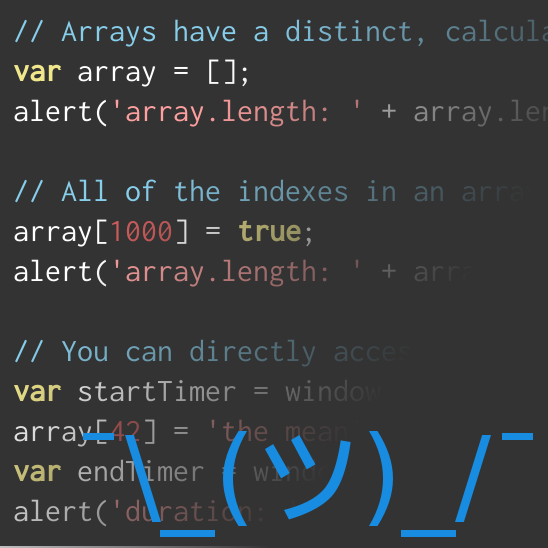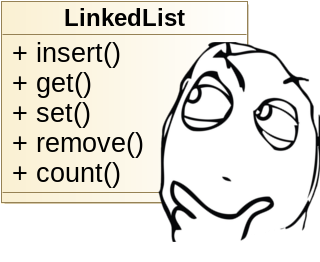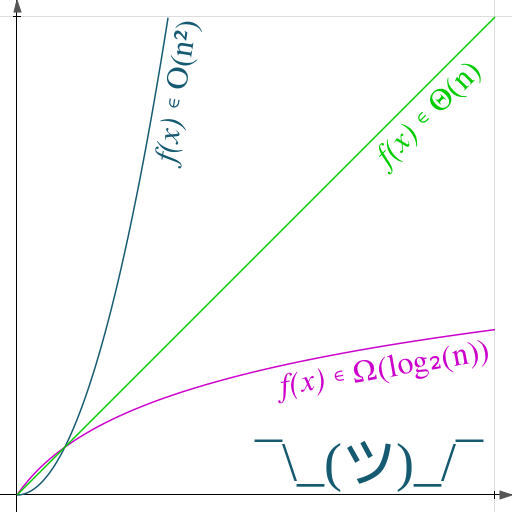## Data Structure: Array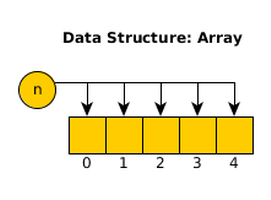Different programming languages have different interpretations of what constitutes an array — indeed, many languages don't even implement "true" arrays in a way that allow direct access by developers. JavaScript and PHP (for example) have data structures that are semantically equivalent to arrays, but they do not exhibit the key features that make arrays unique or special. If, however, you were working in a language that considers arrays to be a unique and distinct data structures then it behooves you to understand what an array is and what makes it useful.

A "true" array can be most accurately described as a series of "things". The "length" of each index is known in advance because each entity is of the same data type. Since the array tracks its own starting address, jumping to a random index in an array simply requires us to calculate the formula start + (index · length) to find the memory address of the requested element. This translates to a constant-time implementation: $\Theta(1)$.

In other words, it doesn't matter what element you want to retrieve from an array, it's going to be extremely fast. This stands in stark contrast to the same behavior from a Linked List. Unless the linked list has been explicitly optimized for random access (which the linked example is not), then random access will be directly related to the index of the element being accessed. Since the list must start at one end of the list and iterate until it gets to the indicated node, random access on a linked list executes in linear time: $\Theta(n)$. At very low numbers, the difference is insignificant; but if you need to randomly access elements of a 100-million index array versus a 100-million index linked-list with any regularity, then the difference in speed could become noticeable.

If arrays are so fast, then why even bother with linked lists?

Arrays are only faster than linked lists within a narrow set of circumstances. Outside of those criteria, arrays can actually perform far, far worse than a linked list if you don't take steps to mitigate those circumstances. As an analogy, an array is like a sheet of lined paper but a linked list is like a stack of flashcards. A sheet of lined paper is quicker to fill out and it takes up less space than a stack of flash cards; but if you ever need to shuffle your cards, you don't need to write them all out again.

### The Pros

The first advantage of using an array rather than a linked list is that an array consume less memory. A linked list requires nodes to carry values and positional data. By contrast, the values in an array are mapped directly to memory addresses. This saves us space in memory for the data structure and the nodes.

// creates an array that holds 30 integers
int myArray;

The second benefit is that traversing arrays incurs a trivial cost, regardless of the jump between indexes. Consider the example of iterating from the last element in an array to the first element in the array. This relatively common requirement incurs a non-trivial overhead in singly linked lists that have not been specifically optimized for this behavior. If we were to use the 30-element array from earlier, and assume we're using this implementation of the Singly Linked List, then this code would follow this pattern:

for (int i = 30; i-- > 0; i) {
myList.get(i); // do things
myArray[i]; // do other things
}


This is simple enough on the surface, but it hides the underlying costs of the linked list. Accessing an element in the array runs at $\Theta(1)$, and we are doing it $n$ times for a total of $\Theta(n)$. Accessing an element in a linked list with the get(x) function must iterate through elements 0 through x. This means that we'd access the xth element once, the (x - 1)th element twice, etc. until the first element is accessed x times. With this pattern, we can model the costs associated with iterating the array:

$f(x) = x + (x - 1) + (x - 2) + ... + 1$ $\equiv \sum_{i = 1}^{x}i \equiv x!$ $\therefore f(x) \in O(n!)$

If you don't understand the math, that's fine; what we've proven is that traversing an array of $n$ elements executes at $\Theta(n)$, while traversing an identical linked list costs us $\Theta(n^2)$. Simply put, iterating an array is way, way faster than iterating a linked list.

### The Cons

There are also some disadvantages to using an array over a linked list. First, some languages (like C/C++) do not allow you to dynamically resize the array. If you ever need to extend the size of your array in these languages, you must first create a new array and copy all of your values into that array. This incurs a cost proportional to the length of the "new" array — $\Theta(n)$.

// copies the contents of an array into a larger array
template<typename T>
T* extendArray(T* oldArray, uint oldLength, uint newLength)
{
T* newArray = new T[newLength];
for (uint i = 0; i < oldLength; ++i) {
newArray[i] = oldArray[i];
}
return newArray;
}

The second major disadvantage is that arrays use static indexing. If you ever need to reindex the elements of an array, then you must do this manually. This can happen any time you insert an element into into (or remove an element from) the beginning or middle of an array, and it forces you to reindex every element that follows. This is a relatively simple operation to conceptualize, but it's slower than the $\Theta(1)$ cost of a Linked List:

// inserting a value in the middle of an array:
template<typename T>
void insert(T* array, uint length, uint index, T value)
{
// extend the existing array by 1
array = extendArray(array, length, length + 1);
while (length > index) {
array[length] = array[--length];
}
array[index] = value;
}

This is an example implementation, but it details the complexity in shifting indexes around. The closer to the "front" of an array that the insertion is performed, the worse the function performs. The best that this operation can perform is against an upper-limit of $O(n)$, depending on the size of the array and where the insertion or deletion is made.

A linked list also suffers from these shortcomings, but with opposite effect. A linked list experiences the best insertion performance at the head of the data structure. If a data structure has been optimized for this behavior, an array experiences the best insertion performance at the end of an array. These differences make each data structure well suited for very different applications.

### Summary

In general, an array is a great data structure for situations where a developer doesn't need to perform insertions or deletions at random locations, but will need to randomly access elements in the array. Without understanding the strengths that make an array unique and useful, you may find yourself writing code that performs arduously slowly because of it used an inappropriate data structure for a common task. While you could certainly write code that would technically function with a linked list instead of an array, or vice-versa, understanding the differences between the two will help you to make more informed decisions. Informed decisions, in turn, contribute to better code and a higher quality product — and who doesn't want to be known for turning out great work?

### Related Blog Posts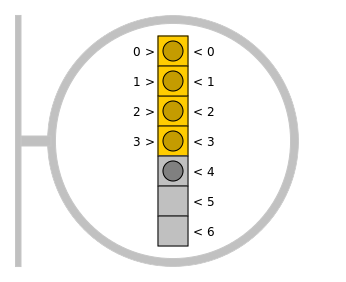Array Lists: A Less Awful Way to Handle Arrays